Скачать презентацию Chp 1 Consumptionbased model and overview 郑振龙 厦门大学金融系

9fd182abba646a87de80d3391ea0fa75.ppt

• Количество слайдов: 52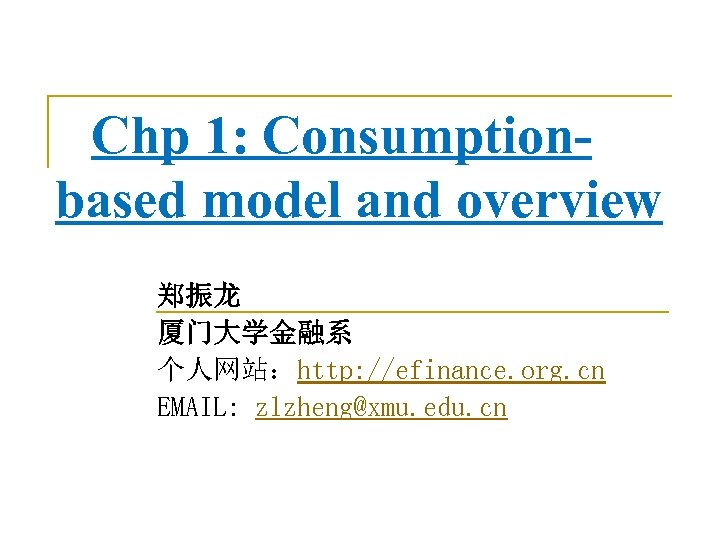Chp 1: Consumptionbased model and overview 郑振龙 厦门大学金融系 个人网站：http: //efinance. org. cn EMAIL: [email protected] edu. cn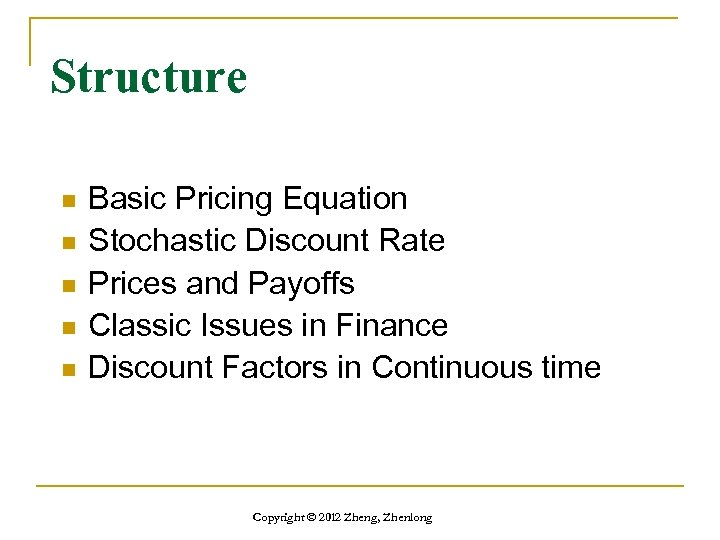Structure n n n Basic Pricing Equation Stochastic Discount Rate Prices and Payoffs Classic Issues in Finance Discount Factors in Continuous time Copyright © 2012 Zheng, Zhenlong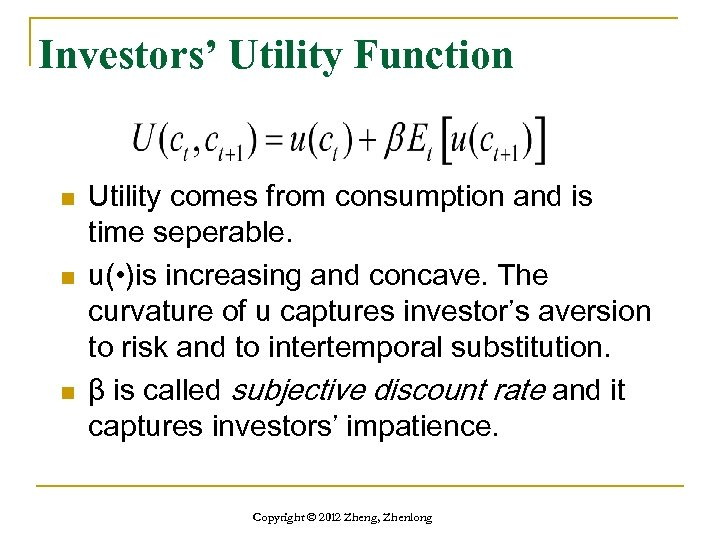Investors’ Utility Function n Utility comes from consumption and is time seperable. u( • )is increasing and concave. The curvature of u captures investor’s aversion to risk and to intertemporal substitution. β is called subjective discount rate and it captures investors’ impatience. Copyright © 2012 Zheng, Zhenlong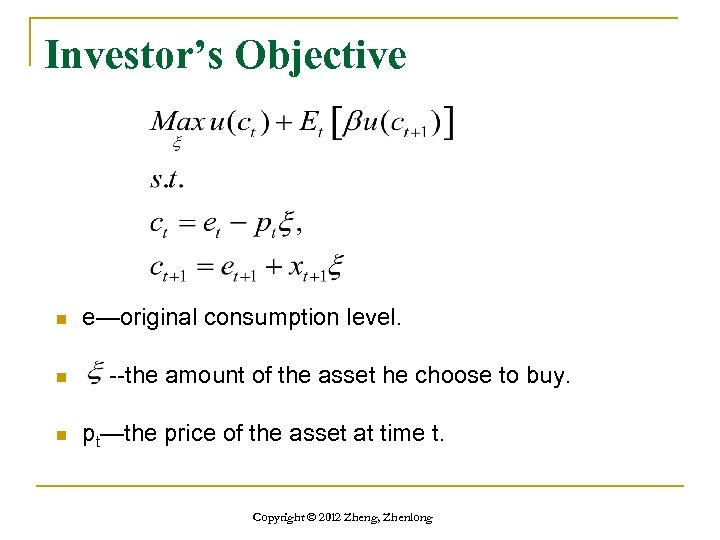Investor’s Objective n n n e—original consumption level. --the amount of the asset he choose to buy. pt—the price of the asset at time t. Copyright © 2012 Zheng, Zhenlong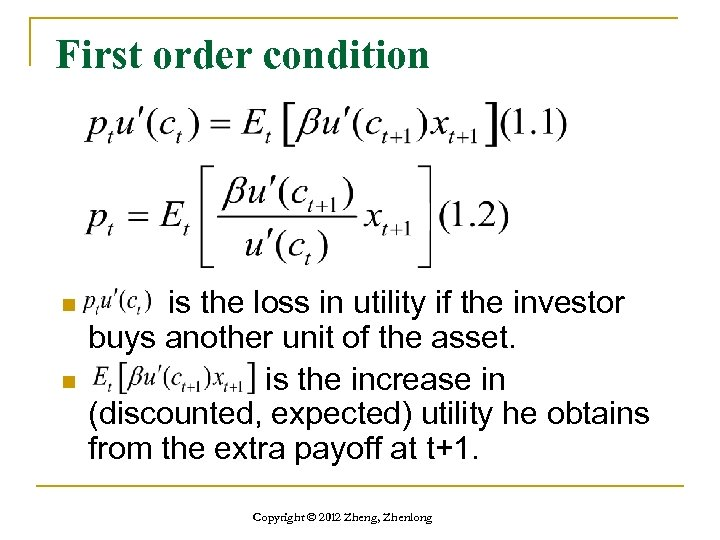First order condition n n is the loss in utility if the investor buys another unit of the asset. is the increase in (discounted, expected) utility he obtains from the extra payoff at t+1. Copyright © 2012 Zheng, Zhenlong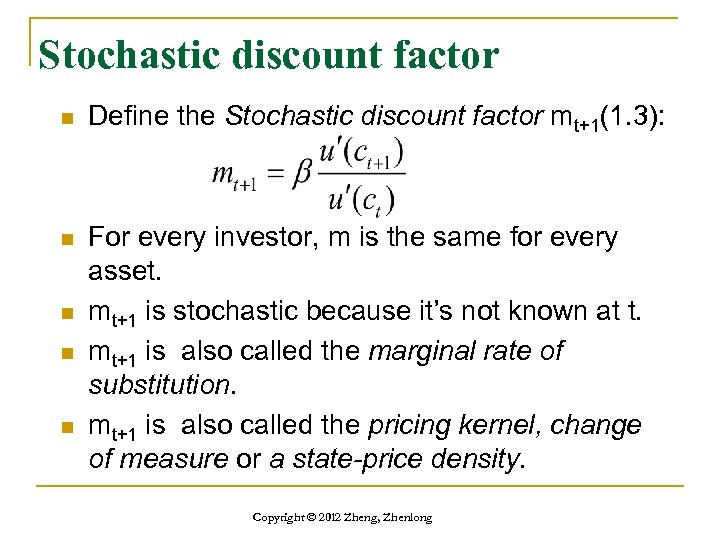Stochastic discount factor n Define the Stochastic discount factor mt+1(1. 3): n For every investor, m is the same for every asset. mt+1 is stochastic because it’s not known at t. mt+1 is also called the marginal rate of substitution. mt+1 is also called the pricing kernel, change of measure or a state-price density. n n n Copyright © 2012 Zheng, Zhenlong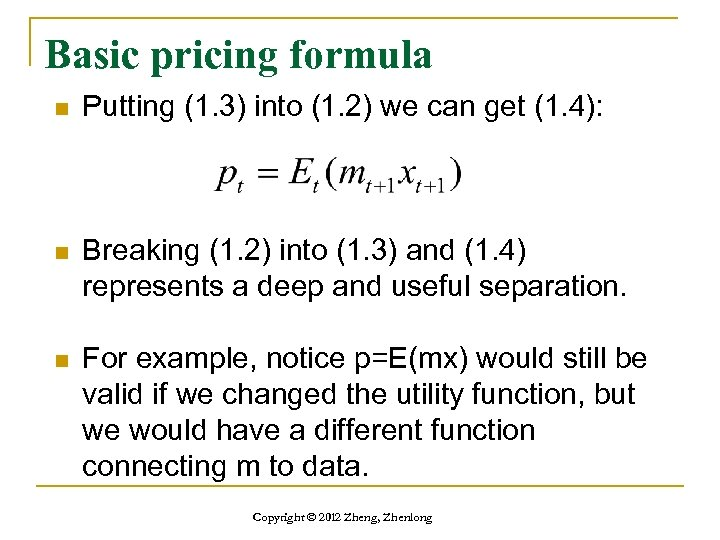Basic pricing formula n Putting (1. 3) into (1. 2) we can get (1. 4): n Breaking (1. 2) into (1. 3) and (1. 4) represents a deep and useful separation. n For example, notice p=E(mx) would still be valid if we changed the utility function, but we would have a different function connecting m to data. Copyright © 2012 Zheng, Zhenlong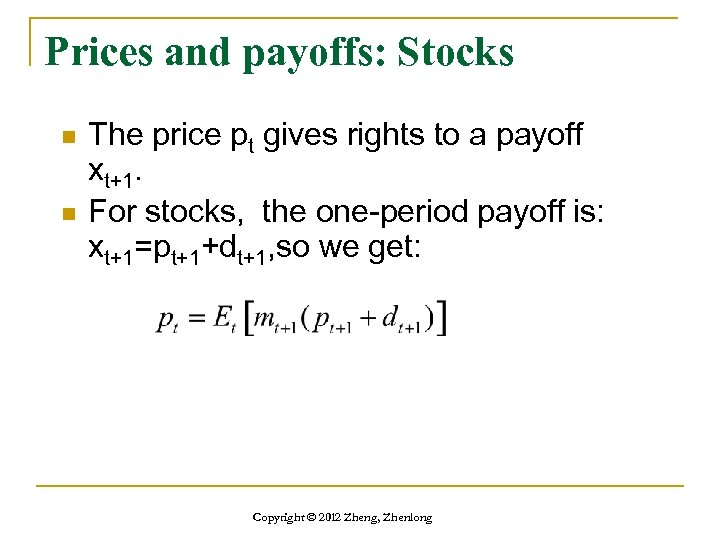Prices and payoffs: Stocks n n The price pt gives rights to a payoff xt+1. For stocks, the one-period payoff is: xt+1=pt+1+dt+1, so we get: Copyright © 2012 Zheng, Zhenlong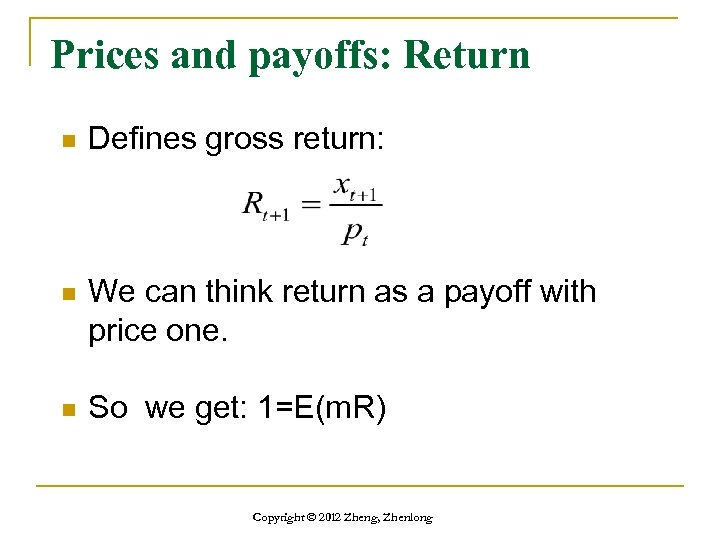Prices and payoffs: Return n Defines gross return: n We can think return as a payoff with price one. n So we get: 1=E(m. R) Copyright © 2012 Zheng, Zhenlong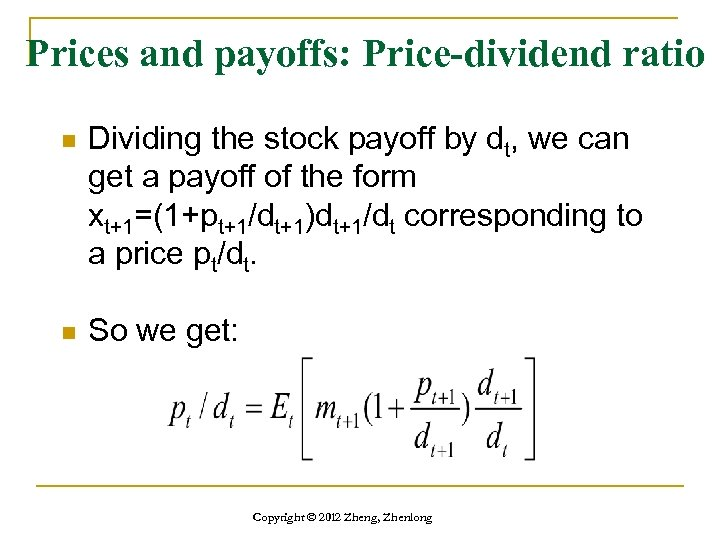Prices and payoffs: Price-dividend ratio n Dividing the stock payoff by dt, we can get a payoff of the form xt+1=(1+pt+1/dt+1)dt+1/dt corresponding to a price pt/dt. n So we get: Copyright © 2012 Zheng, Zhenlong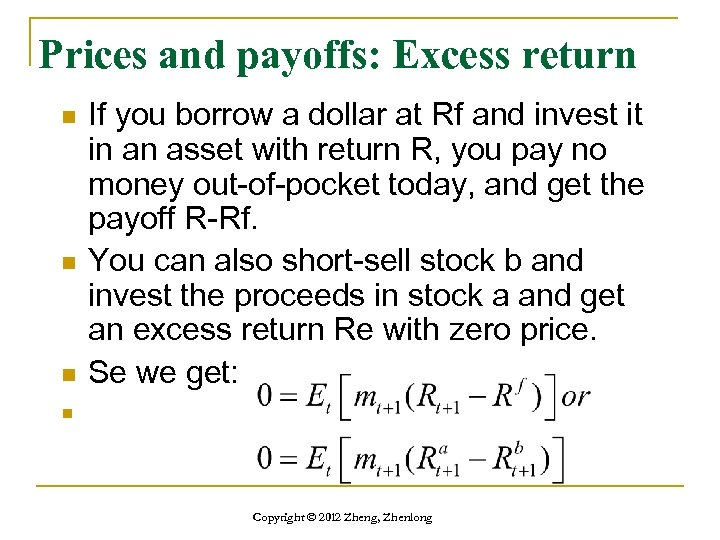Prices and payoffs: Excess return n If you borrow a dollar at Rf and invest it in an asset with return R, you pay no money out-of-pocket today, and get the payoff R-Rf. You can also short-sell stock b and invest the proceeds in stock a and get an excess return Re with zero price. Se we get: n Copyright © 2012 Zheng, Zhenlong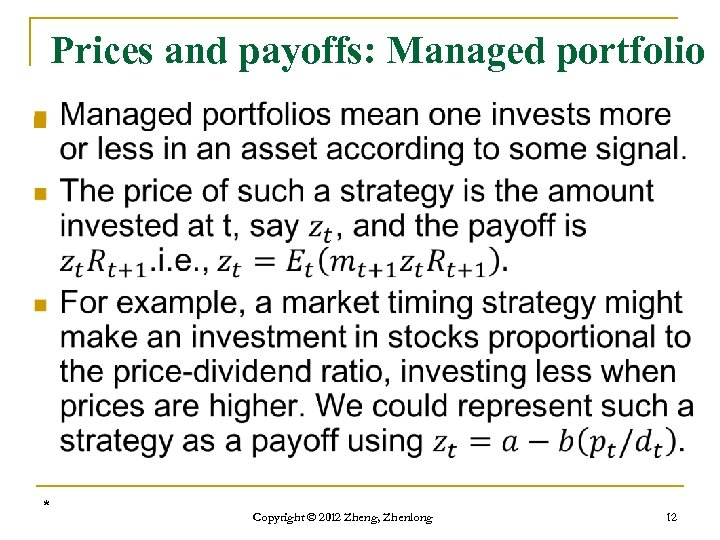Prices and payoffs: Managed portfolio n * Copyright © 2012 Zheng, Zhenlong 12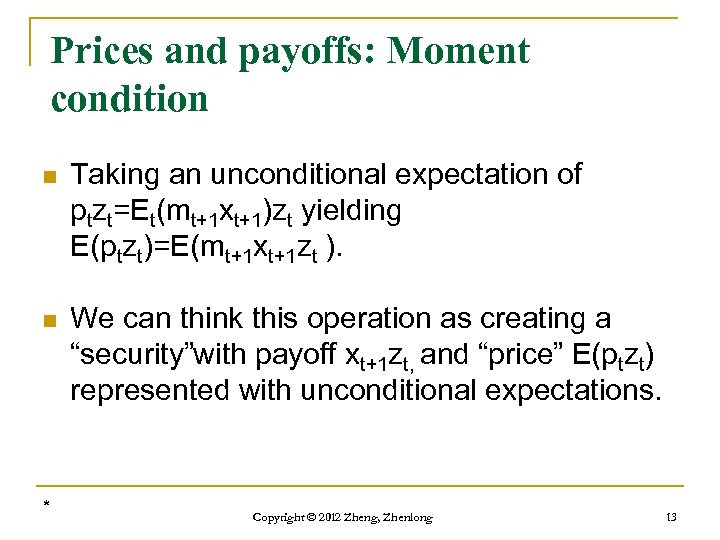Prices and payoffs: Moment condition n Taking an unconditional expectation of ptzt=Et(mt+1 xt+1)zt yielding E(ptzt)=E(mt+1 xt+1 zt ). n We can think this operation as creating a “security”with payoff xt+1 zt, and “price” E(ptzt) represented with unconditional expectations. * Copyright © 2012 Zheng, Zhenlong 13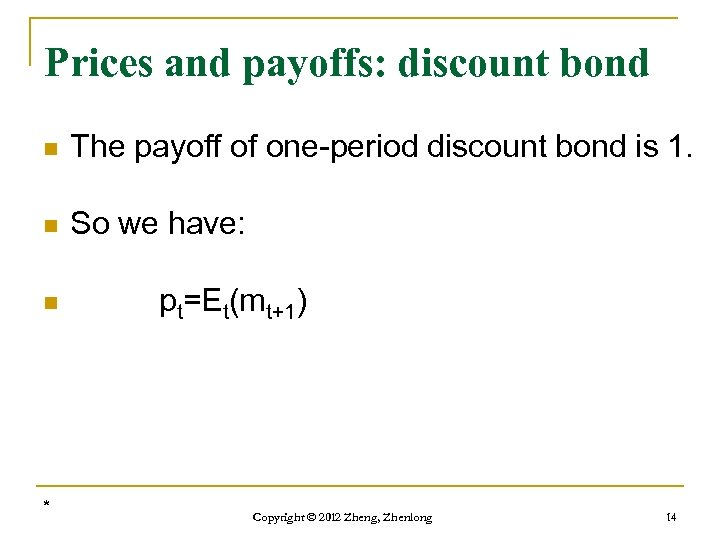Prices and payoffs: discount bond n The payoff of one-period discount bond is 1. n So we have: n * pt=Et(mt+1) Copyright © 2012 Zheng, Zhenlong 14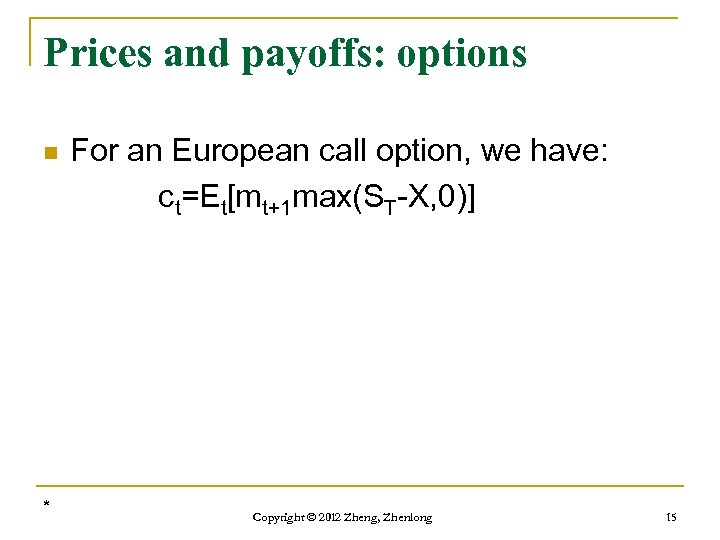Prices and payoffs: options n * For an European call option, we have: ct=Et[mt+1 max(ST-X, 0)] Copyright © 2012 Zheng, Zhenlong 15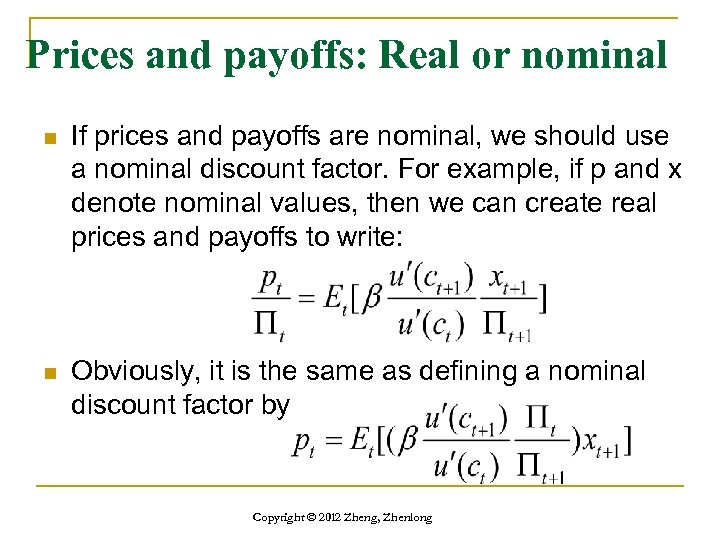Prices and payoffs: Real or nominal n If prices and payoffs are nominal, we should use a nominal discount factor. For example, if p and x denote nominal values, then we can create real prices and payoffs to write: n Obviously, it is the same as defining a nominal discount factor by Copyright © 2012 Zheng, Zhenlong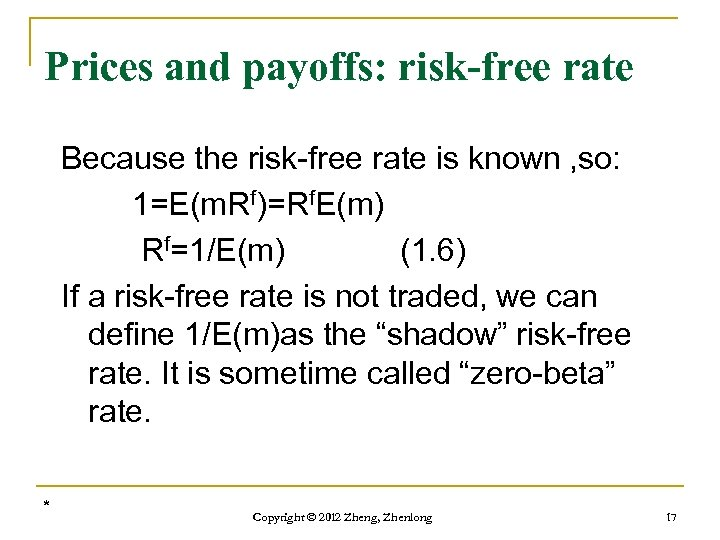Prices and payoffs: risk-free rate Because the risk-free rate is known , so: 1=E(m. Rf)=Rf. E(m) Rf=1/E(m) (1. 6) If a risk-free rate is not traded, we can define 1/E(m)as the “shadow” risk-free rate. It is sometime called “zero-beta” rate. * Copyright © 2012 Zheng, Zhenlong 17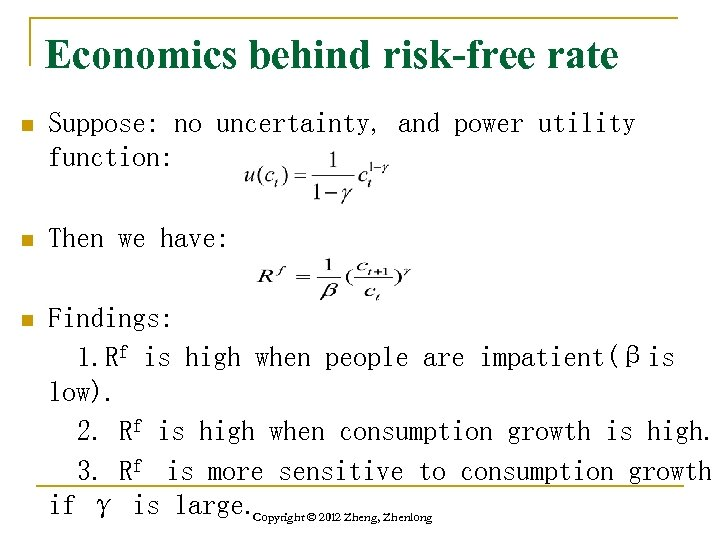Economics behind risk-free rate n Suppose: no uncertainty, and power utility function: n Then we have: n Findings: 1. Rf is high when people are impatient(βis low). 2. Rf is high when consumption growth is high. 3. Rf is more sensitive to consumption growth if γ is large. Copyright © 2012 Zheng, Zhenlong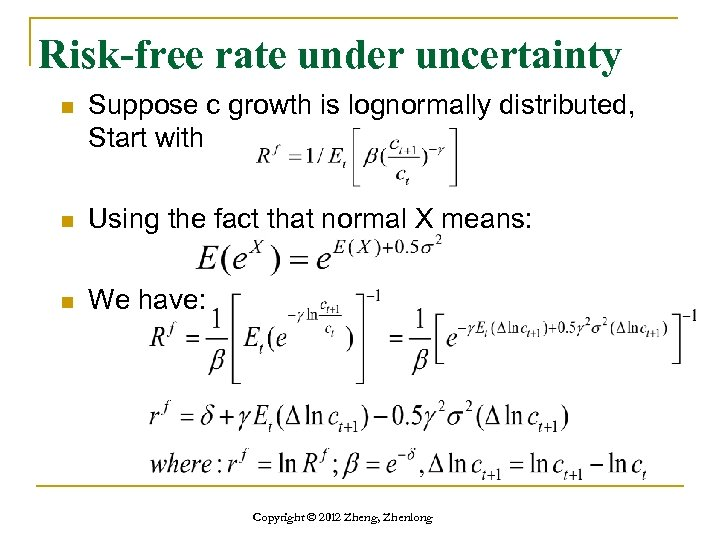Risk-free rate under uncertainty n Suppose c growth is lognormally distributed, Start with n Using the fact that normal X means: n We have: Copyright © 2012 Zheng, Zhenlong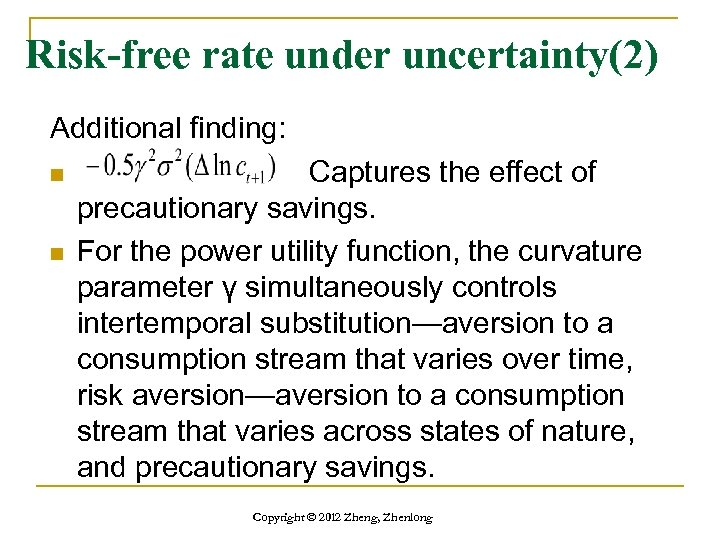Risk-free rate under uncertainty(2) Additional finding: n n Captures the effect of precautionary savings. For the power utility function, the curvature parameter γ simultaneously controls intertemporal substitution—aversion to a consumption stream that varies over time, risk aversion—aversion to a consumption stream that varies across states of nature, and precautionary savings. Copyright © 2012 Zheng, Zhenlong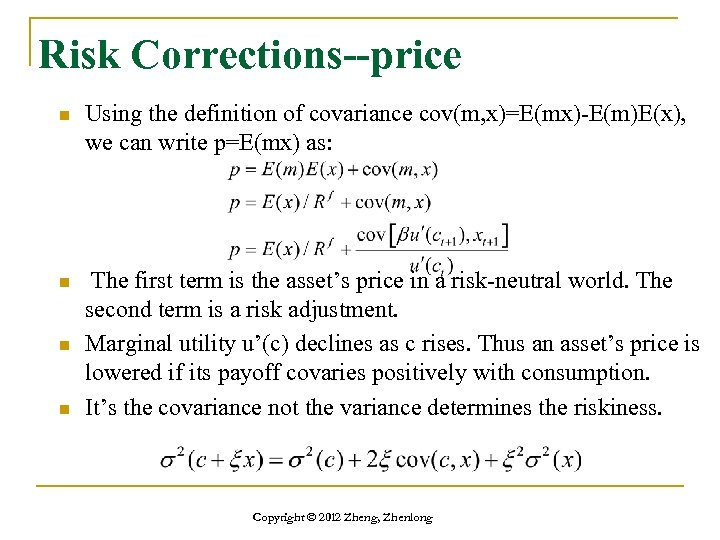Risk Corrections--price n Using the definition of covariance cov(m, x)=E(mx)-E(m)E(x), we can write p=E(mx) as: n The first term is the asset’s price in a risk-neutral world. The second term is a risk adjustment. Marginal utility u’(c) declines as c rises. Thus an asset’s price is lowered if its payoff covaries positively with consumption. It’s the covariance not the variance determines the riskiness. n n Copyright © 2012 Zheng, Zhenlong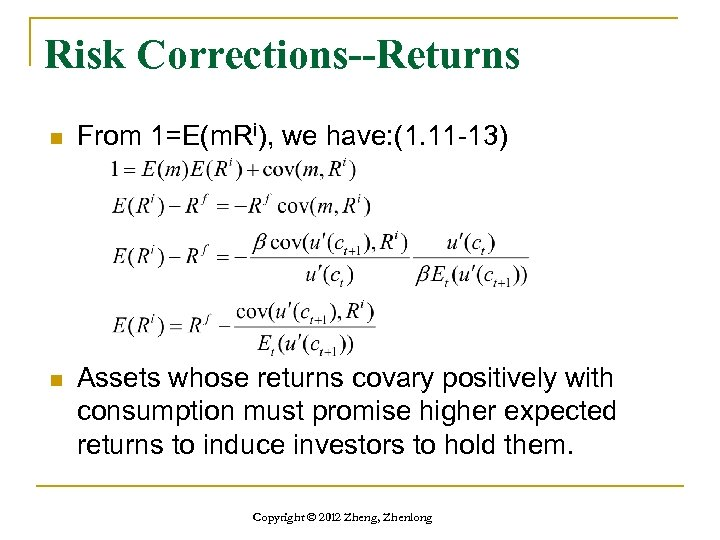Risk Corrections--Returns n From 1=E(m. Ri), we have: (1. 11 -13) n Assets whose returns covary positively with consumption must promise higher expected returns to induce investors to hold them. Copyright © 2012 Zheng, Zhenlong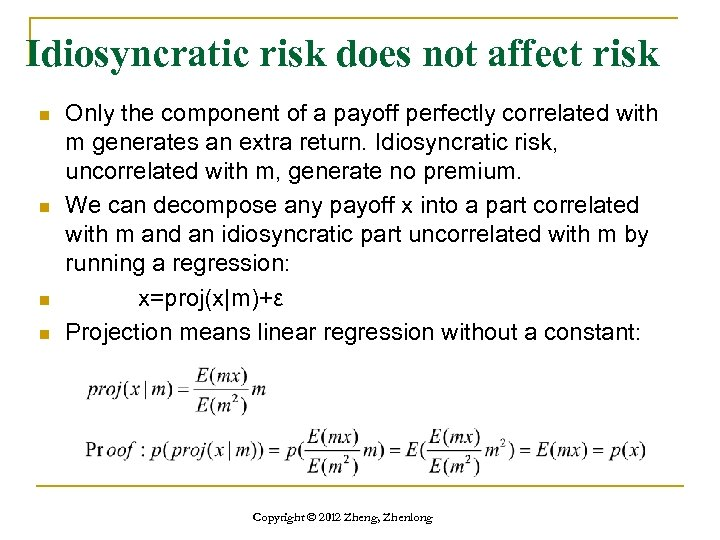Idiosyncratic risk does not affect risk n n Only the component of a payoff perfectly correlated with m generates an extra return. Idiosyncratic risk, uncorrelated with m, generate no premium. We can decompose any payoff x into a part correlated with m and an idiosyncratic part uncorrelated with m by running a regression: x=proj(x|m)+ε Projection means linear regression without a constant: Copyright © 2012 Zheng, Zhenlong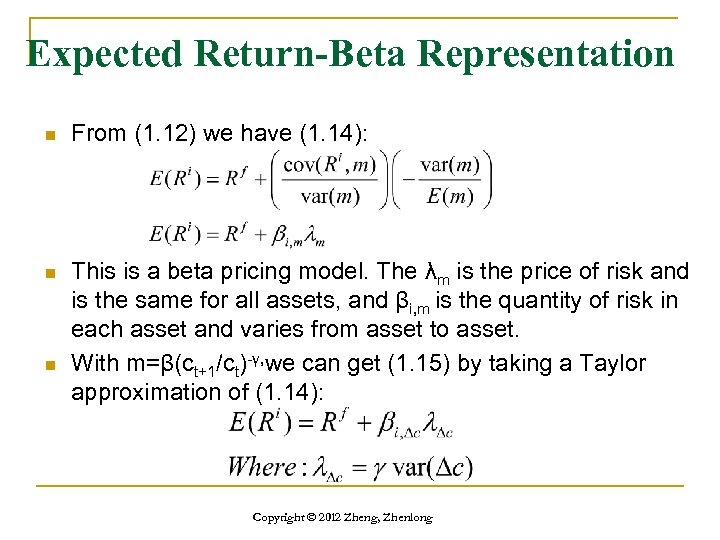Expected Return-Beta Representation n From (1. 12) we have (1. 14): n This is a beta pricing model. The λm is the price of risk and is the same for all assets, and βi, m is the quantity of risk in each asset and varies from asset to asset. With m=β(ct+1/ct)-γ, we can get (1. 15) by taking a Taylor approximation of (1. 14): n Copyright © 2012 Zheng, Zhenlong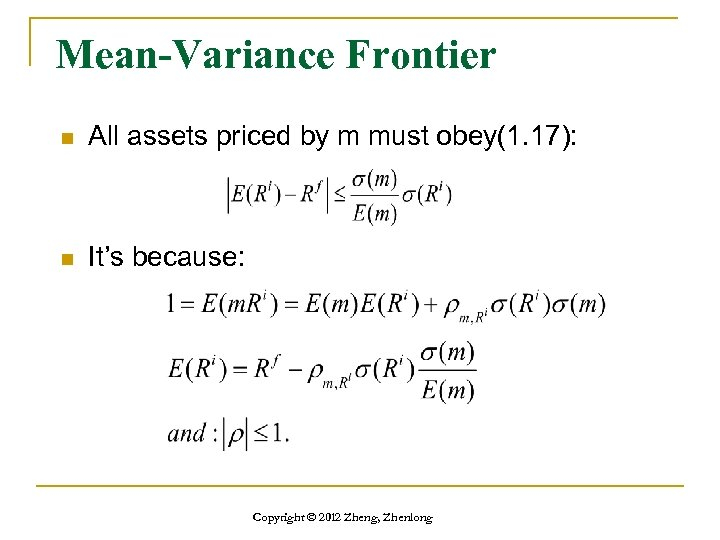Mean-Variance Frontier n All assets priced by m must obey(1. 17): n It’s because: Copyright © 2012 Zheng, Zhenlong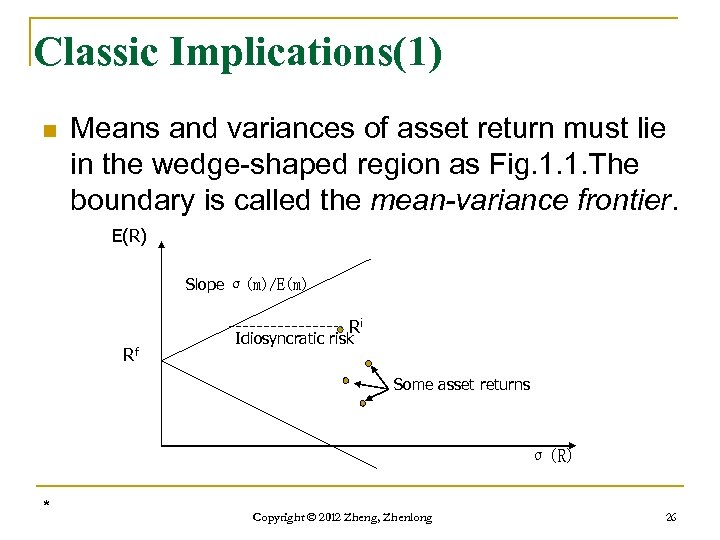Classic Implications(1) n Means and variances of asset return must lie in the wedge-shaped region as Fig. 1. 1. The boundary is called the mean-variance frontier. E(R) Slope σ(m)/E(m) Ri Rf Idiosyncratic risk Some asset returns σ(R) * Copyright © 2012 Zheng, Zhenlong 26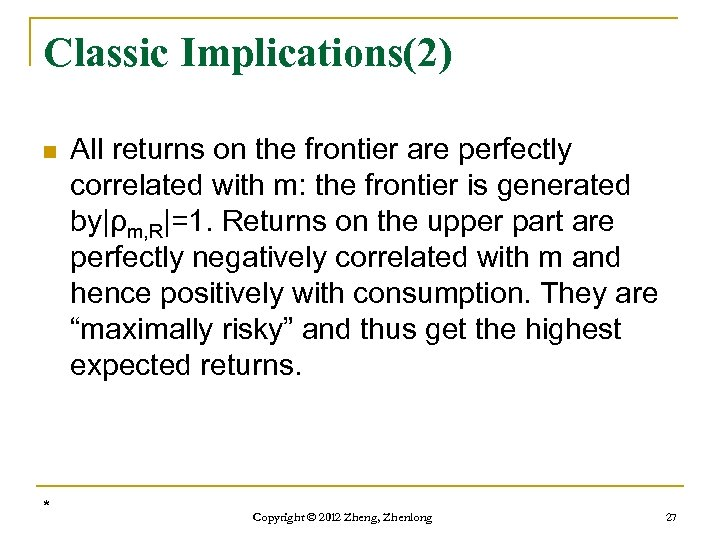Classic Implications(2) n * All returns on the frontier are perfectly correlated with m: the frontier is generated by|ρm, R|=1. Returns on the upper part are perfectly negatively correlated with m and hence positively with consumption. They are “maximally risky” and thus get the highest expected returns. Copyright © 2012 Zheng, Zhenlong 27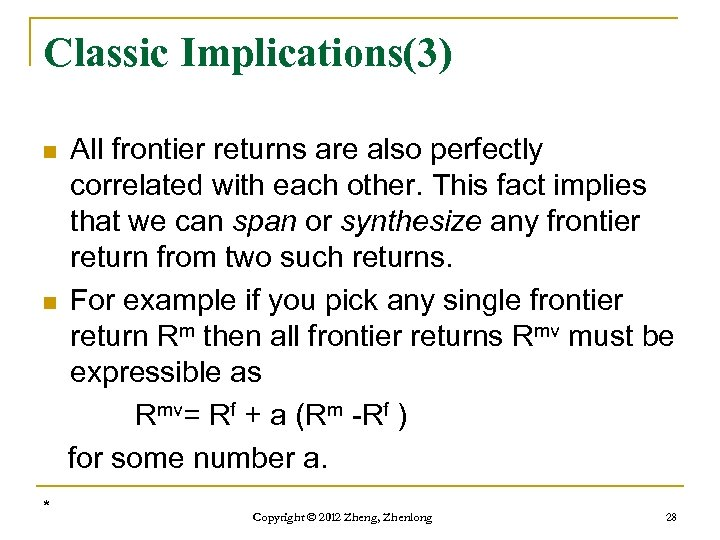Classic Implications(3) n n * All frontier returns are also perfectly correlated with each other. This fact implies that we can span or synthesize any frontier return from two such returns. For example if you pick any single frontier return Rm then all frontier returns Rmv must be expressible as Rmv= Rf + a (Rm -Rf ) for some number a. Copyright © 2012 Zheng, Zhenlong 28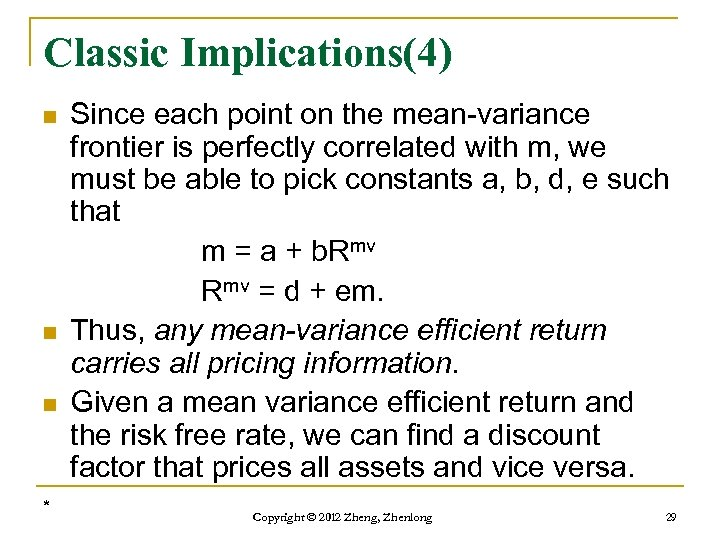Classic Implications(4) n n n * Since each point on the mean-variance frontier is perfectly correlated with m, we must be able to pick constants a, b, d, e such that m = a + b. Rmv = d + em. Thus, any mean-variance efficient return carries all pricing information. Given a mean variance efficient return and the risk free rate, we can find a discount factor that prices all assets and vice versa. Copyright © 2012 Zheng, Zhenlong 29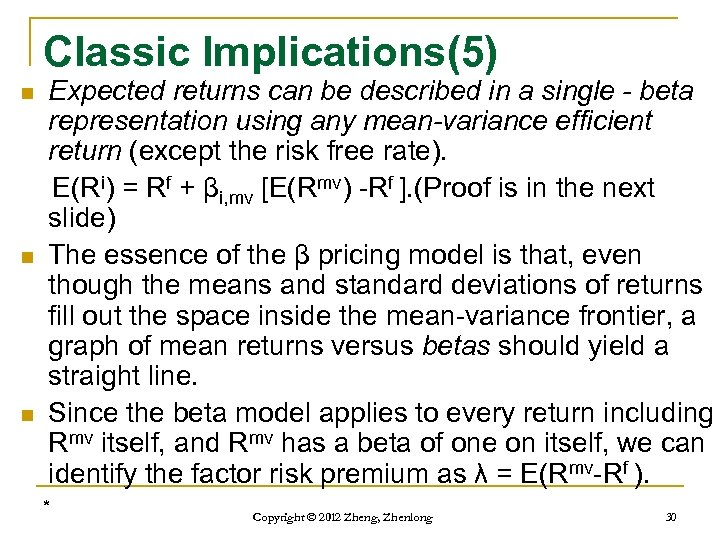Classic Implications(5) n n n Expected returns can be described in a single - beta representation using any mean-variance efficient return (except the risk free rate). E(Ri) = Rf + βi, mv [E(Rmv) -Rf ]. (Proof is in the next slide) The essence of the β pricing model is that, even though the means and standard deviations of returns fill out the space inside the mean-variance frontier, a graph of mean returns versus betas should yield a straight line. Since the beta model applies to every return including Rmv itself, and Rmv has a beta of one on itself, we can identify the factor risk premium as λ = E(Rmv-Rf ). * Copyright © 2012 Zheng, Zhenlong 30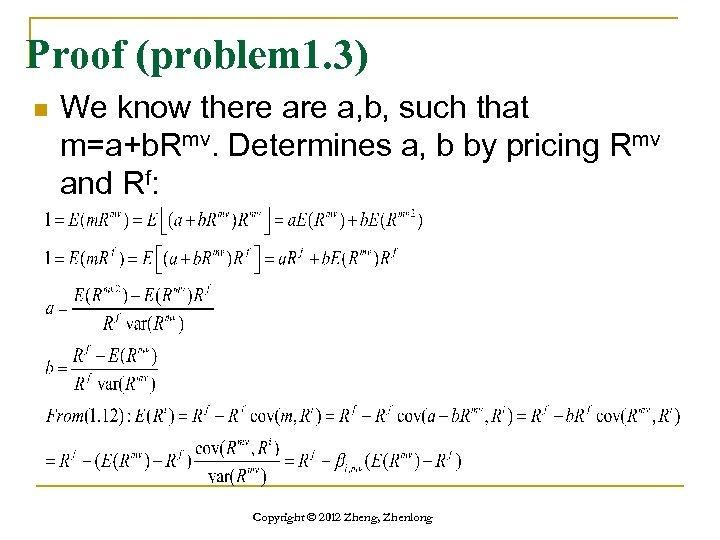Proof (problem 1. 3) n We know there a, b, such that m=a+b. Rmv. Determines a, b by pricing Rmv and Rf: Copyright © 2012 Zheng, Zhenlong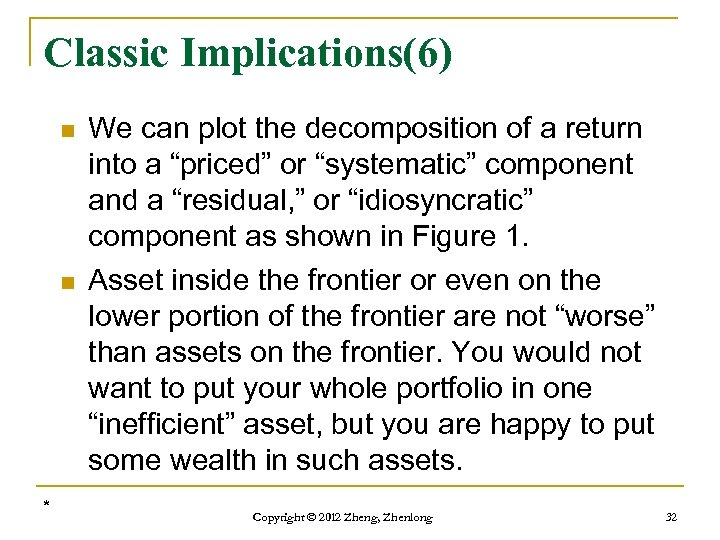Classic Implications(6) n n * We can plot the decomposition of a return into a “priced” or “systematic” component and a “residual, ” or “idiosyncratic” component as shown in Figure 1. Asset inside the frontier or even on the lower portion of the frontier are not “worse” than assets on the frontier. You would not want to put your whole portfolio in one “inefficient” asset, but you are happy to put some wealth in such assets. Copyright © 2012 Zheng, Zhenlong 32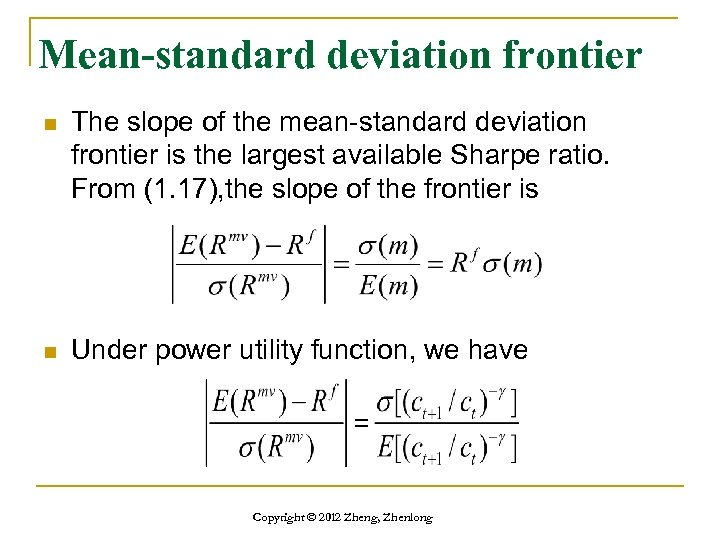Mean-standard deviation frontier n The slope of the mean-standard deviation frontier is the largest available Sharpe ratio. From (1. 17), the slope of the frontier is n Under power utility function, we have Copyright © 2012 Zheng, Zhenlong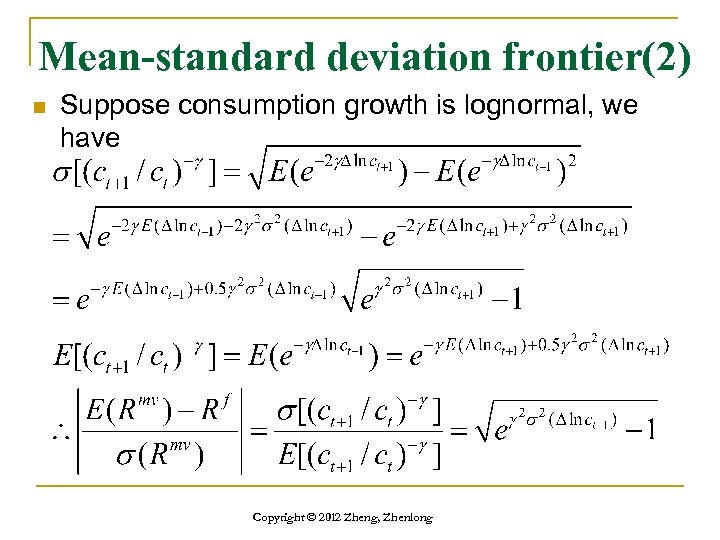Mean-standard deviation frontier(2) n Suppose consumption growth is lognormal, we have Copyright © 2012 Zheng, Zhenlong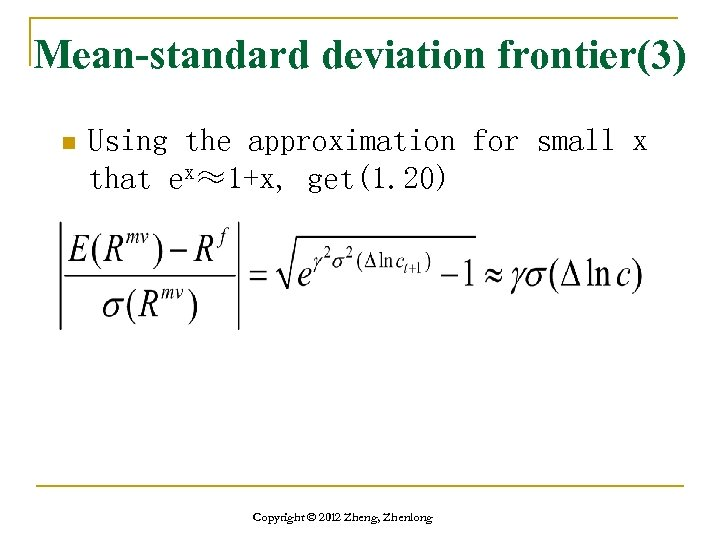Mean-standard deviation frontier(3) n Using the approximation for small x that ex≈1+x, get(1. 20) Copyright © 2012 Zheng, Zhenlong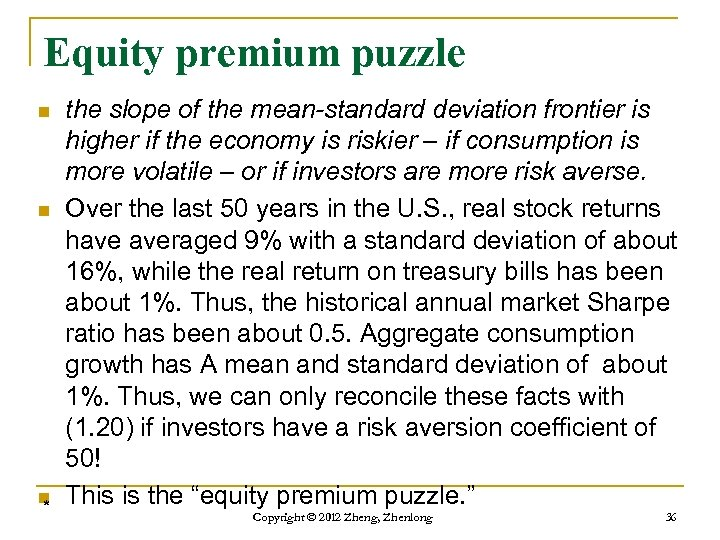Equity premium puzzle n n n * the slope of the mean-standard deviation frontier is higher if the economy is riskier – if consumption is more volatile – or if investors are more risk averse. Over the last 50 years in the U. S. , real stock returns have averaged 9% with a standard deviation of about 16%, while the real return on treasury bills has been about 1%. Thus, the historical annual market Sharpe ratio has been about 0. 5. Aggregate consumption growth has A mean and standard deviation of about 1%. Thus, we can only reconcile these facts with (1. 20) if investors have a risk aversion coefficient of 50! This is the “equity premium puzzle. ” Copyright © 2012 Zheng, Zhenlong 36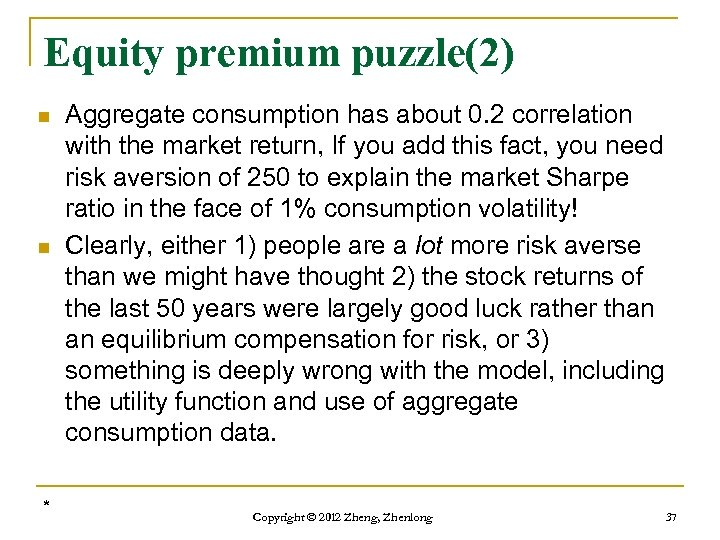Equity premium puzzle(2) n n * Aggregate consumption has about 0. 2 correlation with the market return, If you add this fact, you need risk aversion of 250 to explain the market Sharpe ratio in the face of 1% consumption volatility! Clearly, either 1) people are a lot more risk averse than we might have thought 2) the stock returns of the last 50 years were largely good luck rather than an equilibrium compensation for risk, or 3) something is deeply wrong with the model, including the utility function and use of aggregate consumption data. Copyright © 2012 Zheng, Zhenlong 37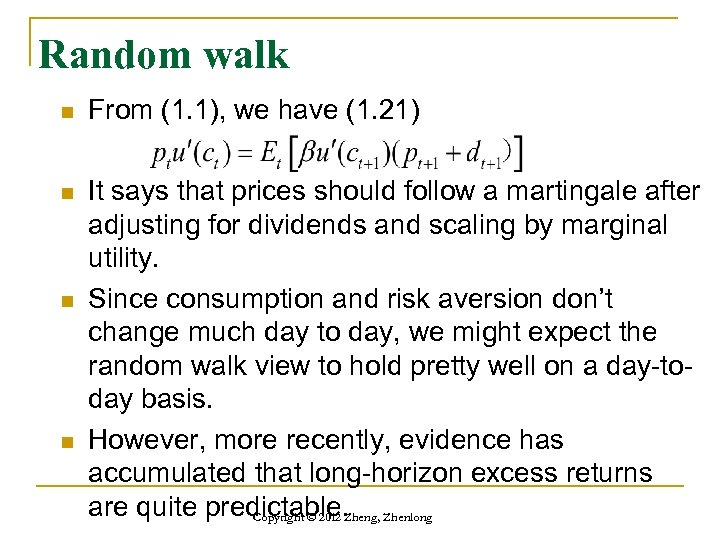Random walk n From (1. 1), we have (1. 21) n It says that prices should follow a martingale after adjusting for dividends and scaling by marginal utility. Since consumption and risk aversion don’t change much day to day, we might expect the random walk view to hold pretty well on a day-today basis. However, more recently, evidence has accumulated that long-horizon excess returns are quite predictable. Zhenlong Copyright © 2012 Zheng, n n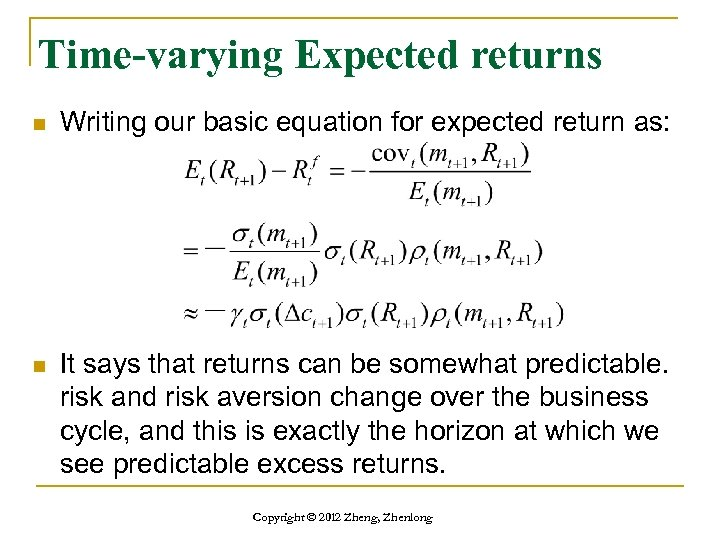Time-varying Expected returns n Writing our basic equation for expected return as: n It says that returns can be somewhat predictable. risk and risk aversion change over the business cycle, and this is exactly the horizon at which we see predictable excess returns. Copyright © 2012 Zheng, Zhenlong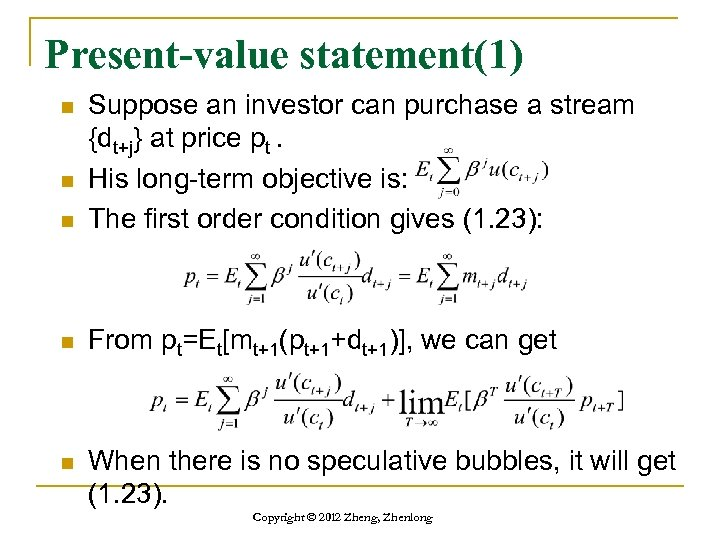Present-value statement(1) n Suppose an investor can purchase a stream {dt+j} at price pt. His long-term objective is: The first order condition gives (1. 23): n From pt=Et[mt+1(pt+1+dt+1)], we can get n When there is no speculative bubbles, it will get (1. 23). n n Copyright © 2012 Zheng, Zhenlong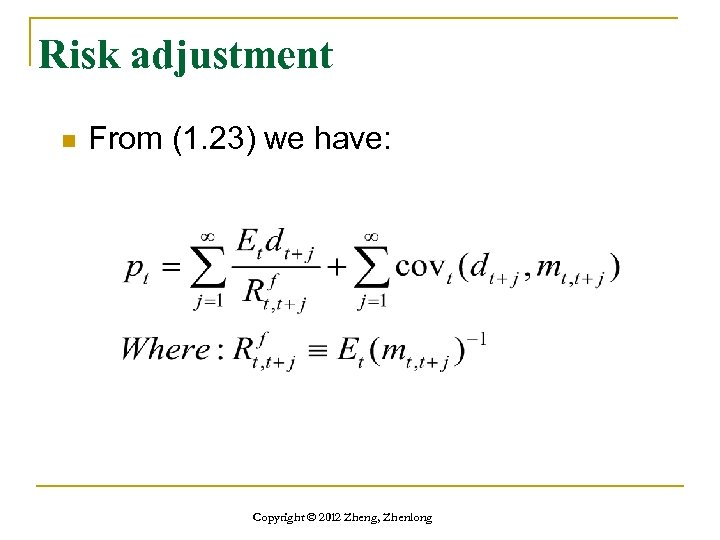Risk adjustment n From (1. 23) we have: Copyright © 2012 Zheng, Zhenlong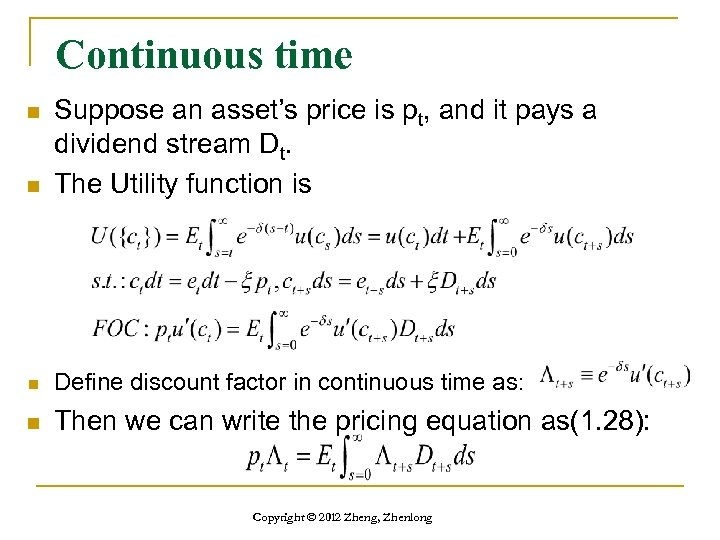Continuous time n Suppose an asset’s price is pt, and it pays a dividend stream Dt. The Utility function is n Define discount factor in continuous time as: n Then we can write the pricing equation as(1. 28): n Copyright © 2012 Zheng, Zhenlong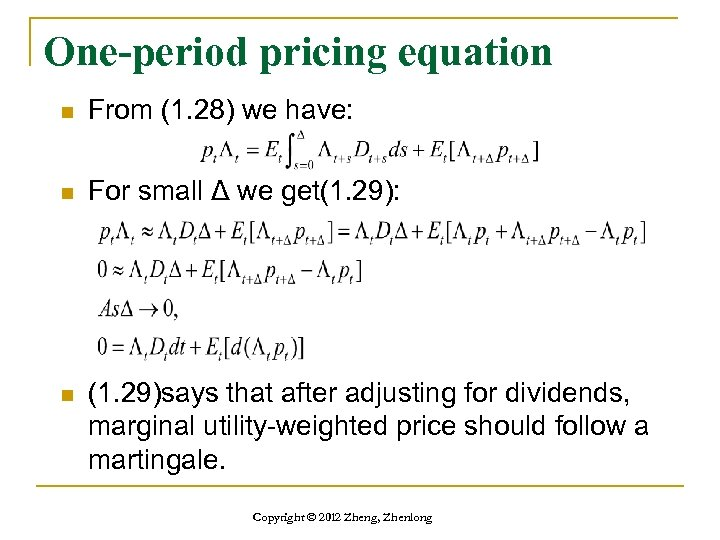One-period pricing equation n From (1. 28) we have: n For small Δ we get(1. 29): n (1. 29)says that after adjusting for dividends, marginal utility-weighted price should follow a martingale. Copyright © 2012 Zheng, Zhenlong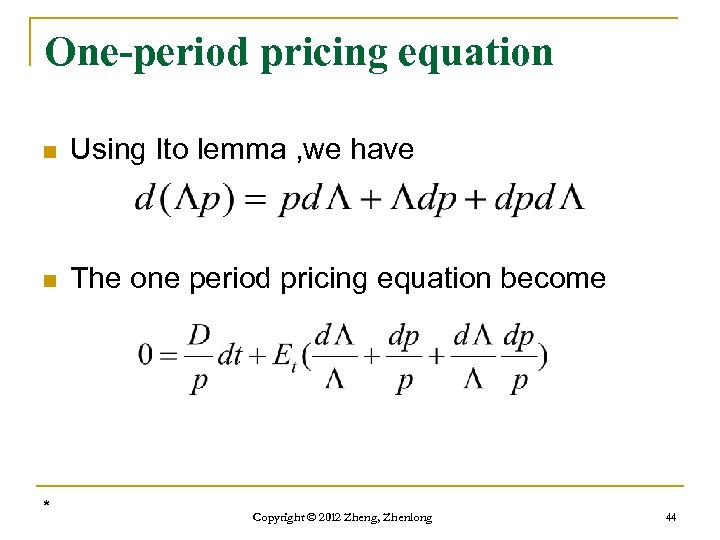One-period pricing equation n Using Ito lemma , we have n The one period pricing equation become * Copyright © 2012 Zheng, Zhenlong 44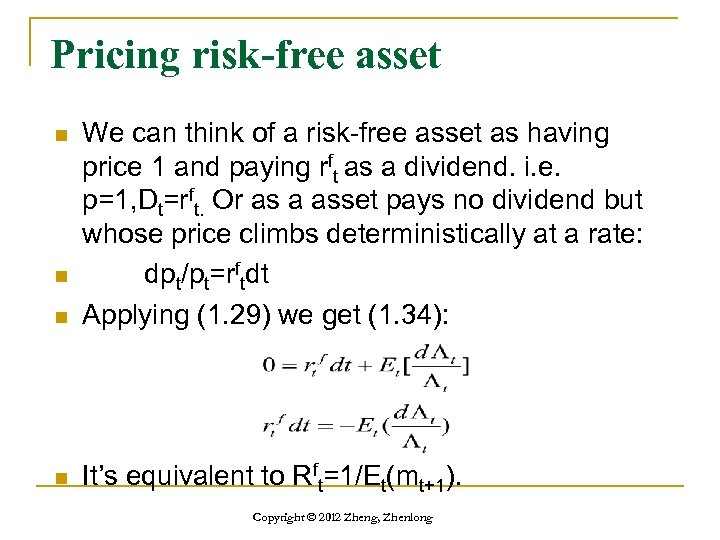Pricing risk-free asset n We can think of a risk-free asset as having price 1 and paying rft as a dividend. i. e. p=1, Dt=rft. Or as a asset pays no dividend but whose price climbs deterministically at a rate: dpt/pt=rftdt Applying (1. 29) we get (1. 34): n It’s equivalent to Rft=1/Et(mt+1). n n Copyright © 2012 Zheng, Zhenlong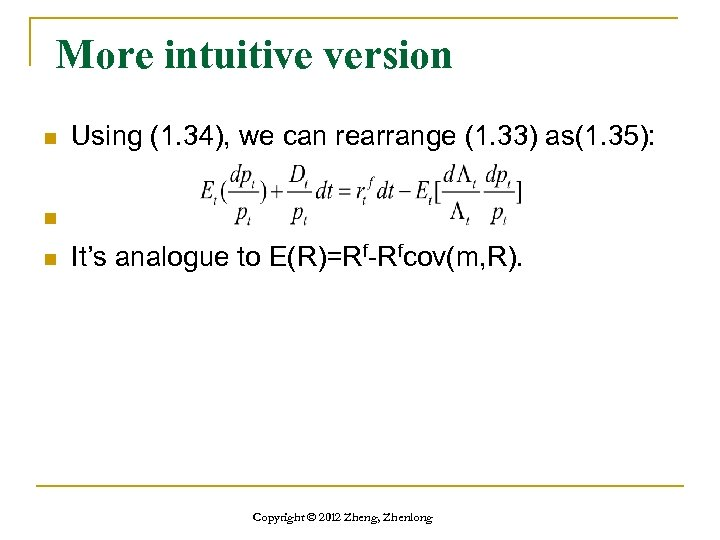More intuitive version n Using (1. 34), we can rearrange (1. 33) as(1. 35): n n It’s analogue to E(R)=Rf-Rfcov(m, R). Copyright © 2012 Zheng, Zhenlong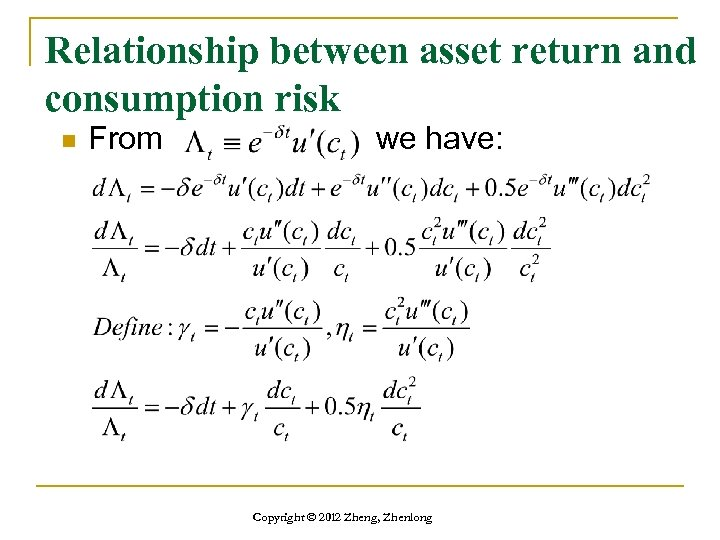Relationship between asset return and consumption risk n From we have: Copyright © 2012 Zheng, Zhenlong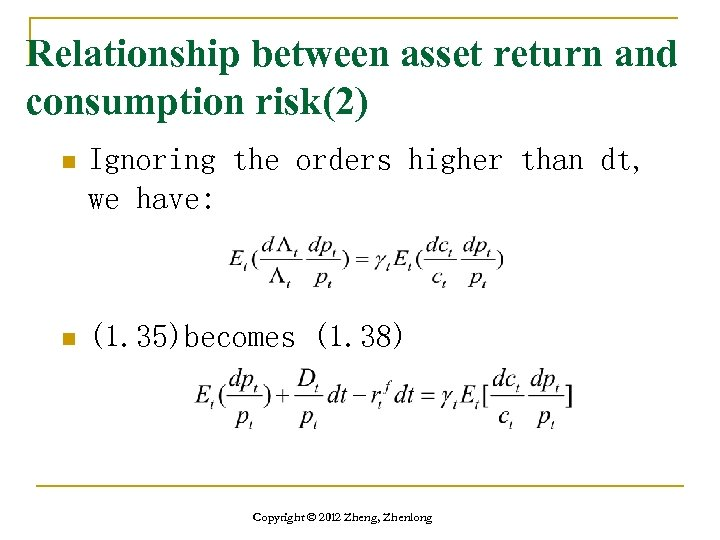Relationship between asset return and consumption risk(2) n Ignoring the orders higher than dt, we have: n (1. 35)becomes (1. 38) Copyright © 2012 Zheng, Zhenlong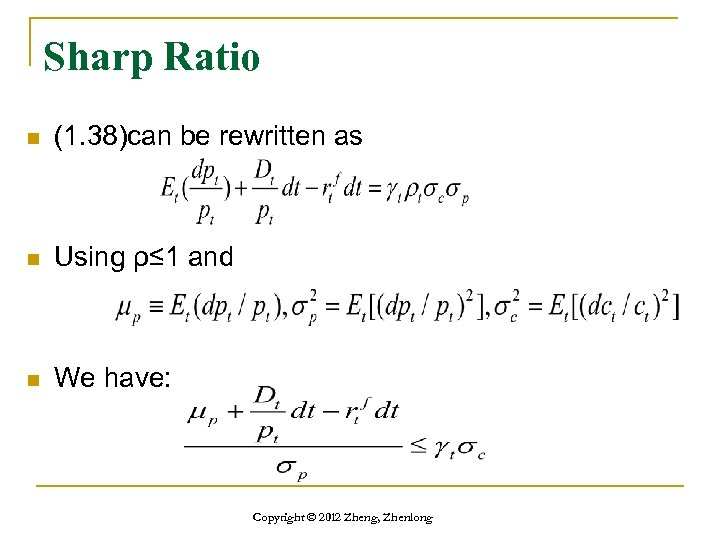Sharp Ratio n (1. 38)can be rewritten as n Using ρ≤ 1 and n We have: Copyright © 2012 Zheng, Zhenlong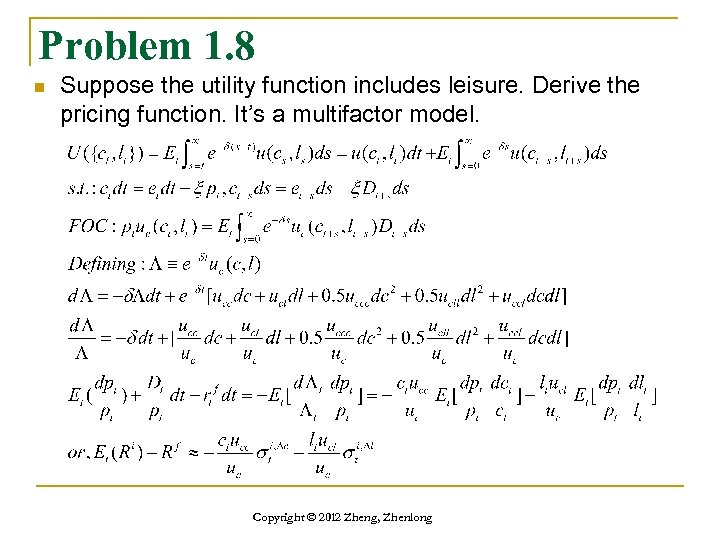Problem 1. 8 n Suppose the utility function includes leisure. Derive the pricing function. It’s a multifactor model. Copyright © 2012 Zheng, Zhenlong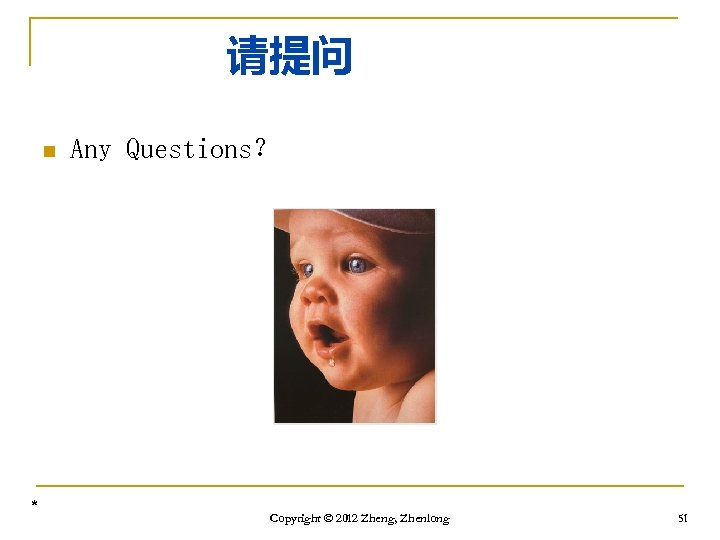请提问 n * Any Questions？ Copyright © 2012 Zheng, Zhenlong 51* Copyright © 2012 Zheng, Zhenlong 52